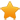Switch Editions?
CancelChannel: CodeSection,代码区,网络安全 - CodeSec
Viewing all articles

# Visualising Residuals

0
0

(This article was first published on blogR , and kindly contributed toR-bloggers)

Residuals. Now there’s something to get you out of bed in the morning!

OK, maybe residuals aren’t the sexiest topic in the world. Still, they’re an essential element and means for identifying potential problems of any statistical model. For example, the residuals from a linear regression model should be homoscedastic . If not, this indicates an issue with the model such as non-linearity in the data.

This post will cover various methods for visualising residuals from regression-based models. Here are some examples of the visualisations that we’ll be creating:

What you need to know

To get the most out of this post, there are a few things you should be aware of. Firstly, if you’re unfamiliar with the meaning of residuals, or what seems to be going on here, I’d recommend that you first do some introductory reading on the topic. Some places to get started are Wikipedia and this excellent section on Statwing .

You’ll also need to be familiar with running regression (linear and logistic) in R, and using the following packages: ggplot2 to produce all graphics, and dplyr and tidyr to do data manipulation. In most cases, you should be able to follow along with each step, but it will help if you’re already familiar with these.

What we’ve got already

Before diving in, it’s good to remind ourselves of the default options that R has for visualising residuals. Most notably, we can directly plot() a fitted regression model. For example, using the mtcars data set, let’s regress the number of miles per gallon for each car ( mpg ) on their horsepower ( hp ) and visualise information about the model and residuals:

fit <- lm(mpg ~ hp, data = mtcars) # Fit the model summary(fit) # Report the results #> #> Call: #> lm(formula = mpg ~ hp, data = mtcars) #> #> Residuals: #> Min 1Q Median 3Q Max #> -5.7121 -2.1122 -0.8854 1.5819 8.2360 #> #> Coefficients: #> Estimate Std. Error t value Pr(>|t|) #> (Intercept) 30.09886 1.63392 18.421 < 2e-16 *** #> hp -0.06823 0.01012 -6.742 1.79e-07 *** #> --- #> Signif. codes: 0 '***' 0.001 '**' 0.01 '*' 0.05 '.' 0.1 ' ' 1 #> #> Residual standard error: 3.863 on 30 degrees of freedom #> Multiple R-squared: 0.6024, Adjusted R-squared: 0.5892 #> F-statistic: 45.46 on 1 and 30 DF, p-value: 1.788e-07 par(mfrow = c(2, 2)) # Split the plotting panel into a 2 x 2 grid plot(fit) # Plot the model information

par(mfrow = c(1, 1)) # Return plotting panel to 1 section

These plots provide a traditional method to interpret residual terms and determine whether there might be problems with our model. We’ll now be thinking about how to supplement these with some alternative (and more visually appealing) graphics.

General Approach

The general approach behind each of the examples that we’ll cover below is to:

Fit a regression model to predict variable (Y). Obtain the predicted and residual values associated with each observation on (Y). Plot the actual and predicted values of (Y) so that they are distinguishable, but connected. Use the residuals to make an aesthetic adjustment (e.g. red colour when residual in very high) to highlight points which are poorly predicted by the model. Simple Linear Regression

We’ll start with simple linear regression, which is when we regress one variable on just one other. We can take the earlier example, where we regressed miles per gallon on horsepower.

Step 1: fit the model

First, we will fit our model. In this instance, let’s copy the mtcars dataset to a new object d so we can manipulate it later:

d <- mtcars fit <- lm(mpg ~ hp, data = d) Step 2: obtain predicted and residual values

Next, we want to get predicted and residual values to add supplementary information to this graph. We can do this as follows:

d\$predicted <- predict(fit) # Save the predicted values d\$residuals <- residuals(fit) # Save the residual values # Quick look at the actual, predicted, and residual values library(dplyr) d %>% select(mpg, predicted, residuals) %>% head() #> mpg predicted residuals #> Mazda RX4 21.0 22.59375 -1.5937500 #> Mazda RX4 Wag 21.0 22.59375 -1.5937500 #> Datsun 710 22.8 23.75363 -0.9536307 #> Hornet 4 Drive 21.4 22.59375 -1.1937500 #> Hornet Sportabout 18.7 18.15891 0.5410881 #> Valiant 18.1 22.93489 -4.8348913

Looking good so far.

Step 3: plot the actual and predicted values

Plotting these values takes a couple of intermediate steps. First, we plot our actual data as follows:

library(ggplot2) ggplot(d, aes(x = hp, y = mpg)) + # Set up canvas with outcome variable on y-axis geom_point() # Plot the actual points

Next, we plot the predicted values in a way that they’re distinguishable from the actual values. For example, let’s change their shape:

ggplot(d, aes(x = hp, y = mpg)) + geom_point() + geom_point(aes(y = predicted), shape = 1) # Add the predicted values

This is on track, but it’s difficult to see how our actual and predicted values are related. Let’s connect the actual data points with their corresponding predicted value using geom_segment() :

Viewing all articles

More Pages to Explore .....